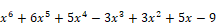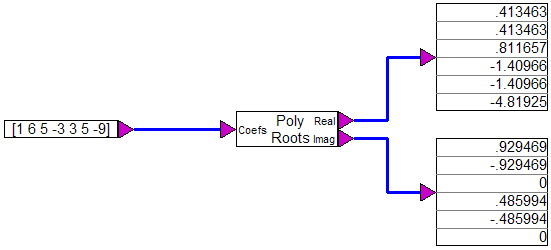## polyRoots

Block Category: Matrix Operation

Description: The polyRoots block finds the roots of polynomials with real coefficients. It accepts one vector input (the coefficients vector, starting with the coefficient of the highest order term at the top), and outputs two vectors (containing the real and corresponding imaginary parts of the roots.

#### Example

The following diagram finds the real and imaginary roots for the polynomial